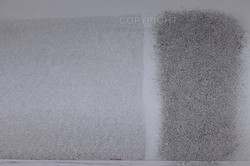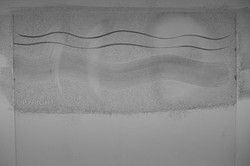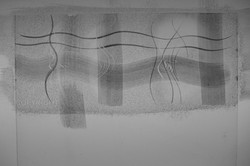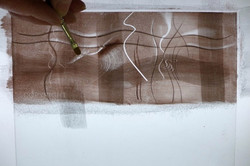Time and Temperature

Figure 1Figure 2

Figure 2 from Jonathan's book "Time and Temperature"Figure 3

Figure 3 from Jonathan's book "Time and Temperature"Figure 4

Figure 4 from Jonathan's book "Time and Temperature"Figure 5

Figure 5 from Jonathan's book "Time and Temperature"Figure 6

Figure 6 from Jonathan's book "Time and Temperature"Figure 7

Figure 7 from Jonathan's book "Time and Temperature"Figure 8

Figure 8 from Jonathan's book "Time and Temperature"Figure 9

Figure 9 from Jonathan's book "Time and Temperature"Figure 10

Figure 10 from Jonathan's book "Time and Temperature"Figure 11

Figure 11 from Jonathan's book "Time and Temperature"Figure 12

Figure 12 from Jonathan's book "Time and Temperature"Figure 13

Figure 13 from Jonathan's book "Time and Temperature"Figure 14

Figure 14 from Jonathan's book "Time and Temperature"Figure 15

Figure 15 from Jonathan's book "Time and Temperature"Figure 16

Figure 16 from Jonathan's book "Time and Temperature"Figure 17

Figure 17 from Jonathan's book "Time and Temperature"